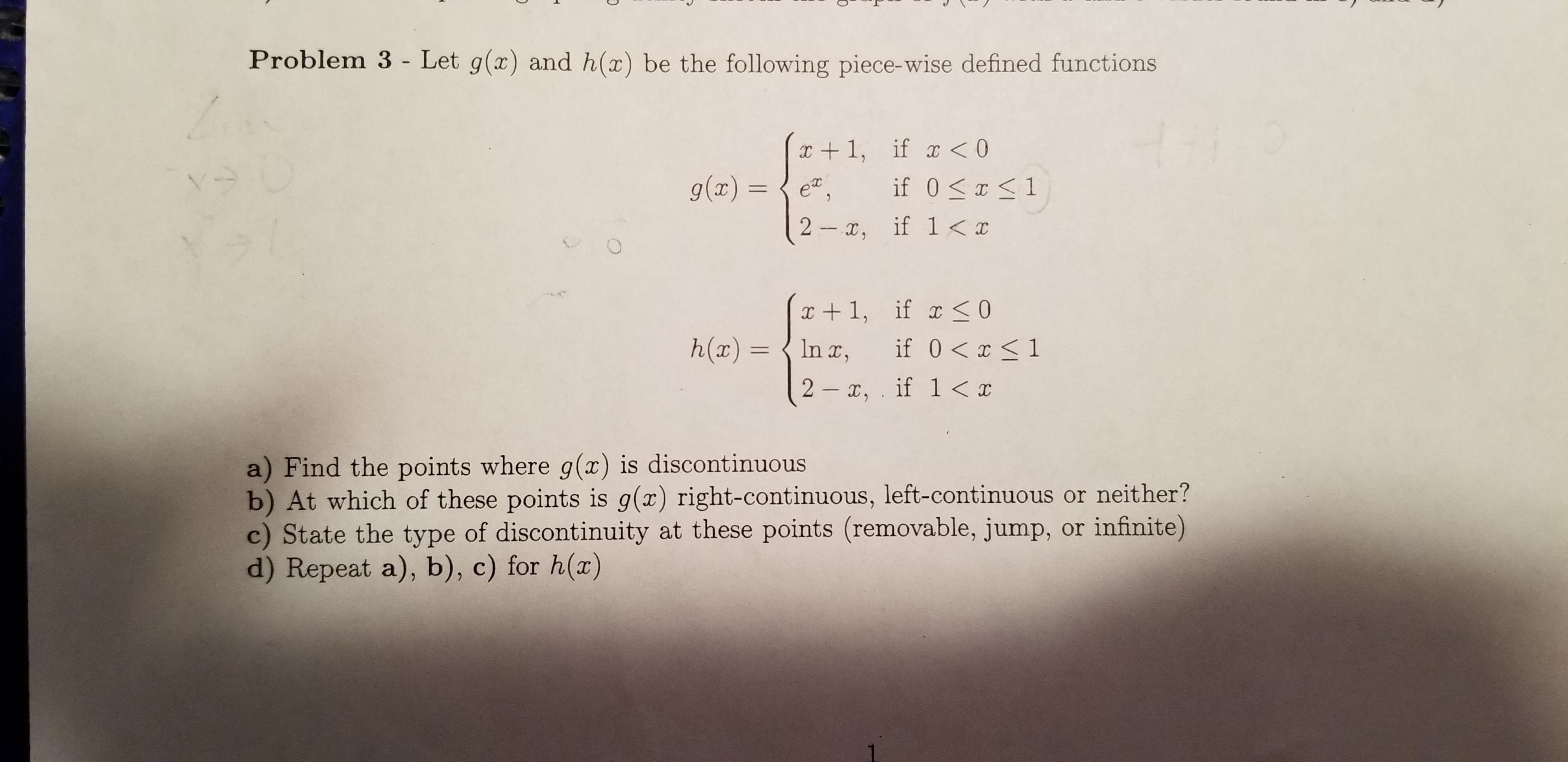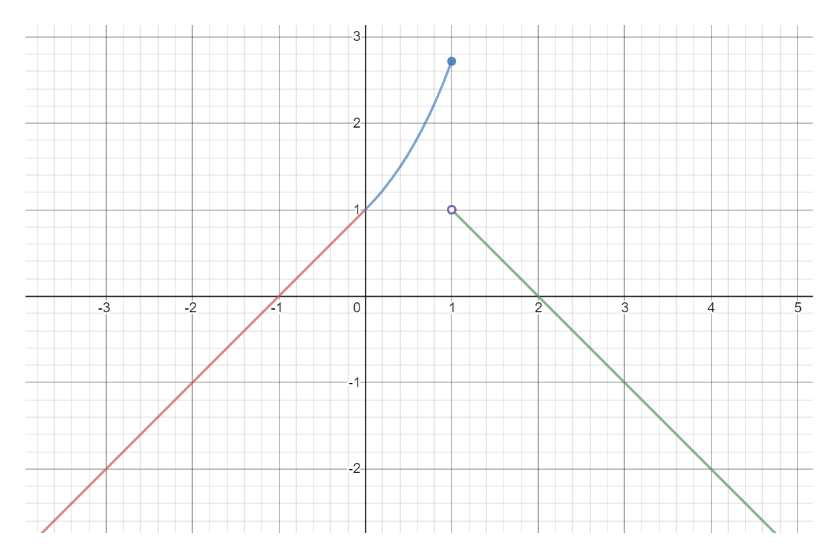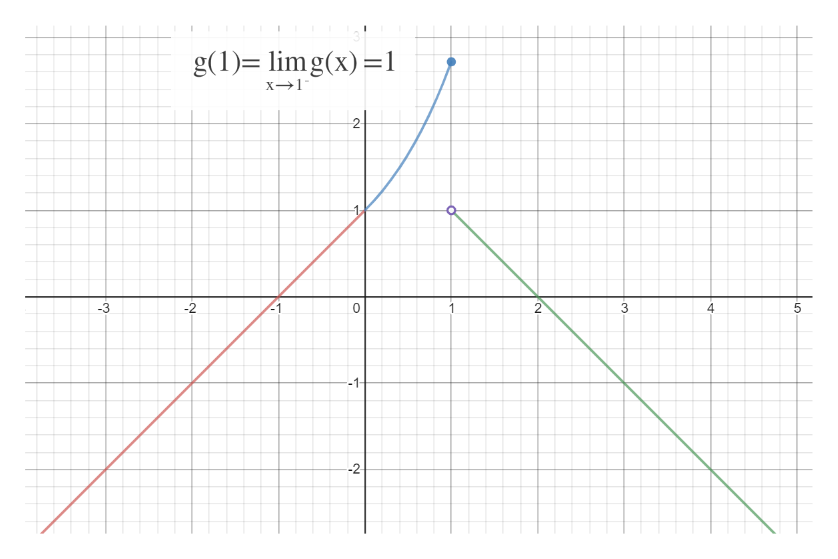Problem 3 - Let g(x) and h(x) be the following piece-wise defined functionsx1, if x

Question

Problem 3 in attached imagehelp_outlineImage TranscriptioncloseProblem 3 - Let g(x) and h(x) be the following piece-wise defined functions x1, if x<0 if 0 1 g(ax) IC 2 , if 1
Step 1

Since we only answer up to 3 sub-parts, we’ll answer the first 3. Please resubmit the question and specify the other subparts (up to 3) you’d like answered.

Possible discontinuities of g(x) are at x=0 and x=1.

We find left and right hand limits at x=0 and x=1.

Step 2

At x=0, left hand limit=0+1=1

And right hand limit = e^0=1

So at x=0, g(x) is continuous.

At x=1 left hand limit=e^1=e

Right hand limit=2-1=1

So, at x=1 the graph is discontinuous.help_outlineImage Transcriptionclose3 2 -3 -2 2 3 -1 -2 LO C fullscreen
Step 3

g(1)= left hand of g(x) limit at x=1help_outlineImage Transcriptioncloseg(1)= lim g(x)1 x1 2 -3 2 C 2 4 --1 -2 fullscreen

Want to see the full answer?

See Solution

Want to see this answer and more?

Our solutions are written by experts, many with advanced degrees, and available 24/7

See Solution
Tagged in

Continuity